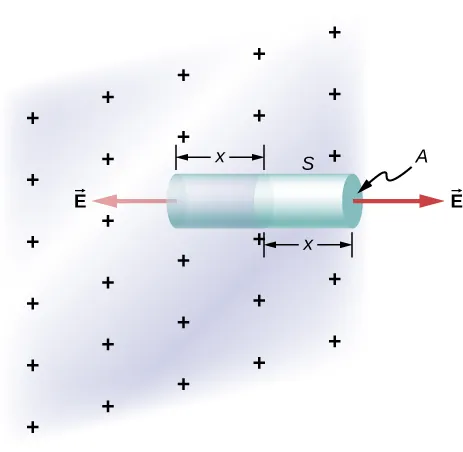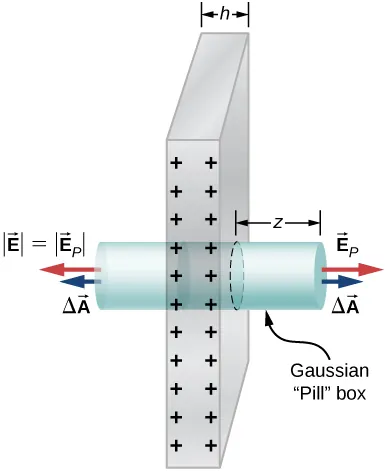University Physics Volume 2

# Challenge Problems

University Physics Volume 2Challenge Problems

### Challenge Problems

91.

The Hubble Space Telescope can measure the energy flux from distant objects such as supernovae and stars. Scientists then use this data to calculate the energy emitted by that object. Choose an interstellar object which scientists have observed the flux at the Hubble with (for example, Vega3), find the distance to that object and the size of Hubble’s primary mirror, and calculate the total energy flux. (Hint: The Hubble intercepts only a small part of the total flux.)

92.

Re-derive Gauss’s law for the gravitational field, with $g→g→$ directed positively outward.

93.

An infinite plate sheet of charge of surface charge density $σσ$ is shown below. What is the electric field at a distance x from the sheet? Compare the result of this calculation with that of worked out in the text.94.

A spherical rubber balloon carries a total charge Q distributed uniformly over its surface. At $t=0t=0$, the radius of the balloon is R. The balloon is then slowly inflated until its radius reaches 2R at the time $t0.t0.$ Determine the electric field due to this charge as a function of time (a) at the surface of the balloon, (b) at the surface of radius R, and (c) at the surface of radius 2R. Ignore any effect on the electric field due to the material of the balloon and assume that the radius increases uniformly with time.

95.

Find the electric field of a large conducting plate containing a net charge q. Let A be area of one side of the plate and h the thickness of the plate (see below). The charge on the metal plate will distribute mostly on the two planar sides and very little on the edges if the plate is thin.### Footnotes

Order a print copy

As an Amazon Associate we earn from qualifying purchases.